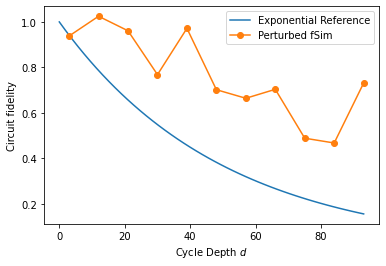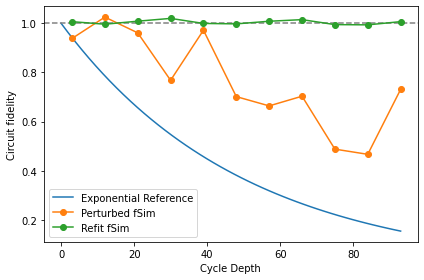XEB and Coherent Error

try:
import cirq
except ImportError:
print("installing cirq...")
!pip install --quiet cirq
print("installed cirq.")
import numpy as np

import cirq
from cirq.contrib.svg import SVGCircuit

Set up Random Circuits

We create a set of 10 random, two-qubit circuits which uses SINGLE_QUBIT_GATES to randomize the circuit and SQRT_ISWAP as the entangling gate. We will ultimately truncate each of these circuits according to cycle_depths. Please see the XEB Theory notebook for more details.

exponents = np.linspace(0, 7/4, 8)
exponents
array([0.  , 0.25, 0.5 , 0.75, 1.  , 1.25, 1.5 , 1.75])
import itertools
SINGLE_QUBIT_GATES = [
cirq.PhasedXZGate(x_exponent=0.5, z_exponent=z, axis_phase_exponent=a)
for a, z in itertools.product(exponents, repeat=2)
]
SINGLE_QUBIT_GATES[:10], '...'
([cirq.PhasedXZGate(axis_phase_exponent=0.0, x_exponent=0.5, z_exponent=0.0),
cirq.PhasedXZGate(axis_phase_exponent=0.0, x_exponent=0.5, z_exponent=0.25),
cirq.PhasedXZGate(axis_phase_exponent=0.0, x_exponent=0.5, z_exponent=0.5),
cirq.PhasedXZGate(axis_phase_exponent=0.0, x_exponent=0.5, z_exponent=0.75),
cirq.PhasedXZGate(axis_phase_exponent=0.0, x_exponent=0.5, z_exponent=1.0),
cirq.PhasedXZGate(axis_phase_exponent=0.0, x_exponent=0.5, z_exponent=1.25),
cirq.PhasedXZGate(axis_phase_exponent=0.0, x_exponent=0.5, z_exponent=1.5),
cirq.PhasedXZGate(axis_phase_exponent=0.0, x_exponent=0.5, z_exponent=1.75),
cirq.PhasedXZGate(axis_phase_exponent=0.25, x_exponent=0.5, z_exponent=0.0),
cirq.PhasedXZGate(axis_phase_exponent=0.25, x_exponent=0.5, z_exponent=0.25)],
'...')
from cirq.experiments import random_quantum_circuit_generation as rqcg

q0, q1 = cirq.LineQubit.range(2)
# Make long circuits (which we will truncate)
n_circuits = 10
circuits = [
rqcg.random_rotations_between_two_qubit_circuit(
q0, q1,
depth=100,
two_qubit_op_factory=lambda a, b, _: cirq.SQRT_ISWAP(a, b),
single_qubit_gates=SINGLE_QUBIT_GATES)
for _ in range(n_circuits)
]
# We will truncate to these lengths
max_depth = 100
cycle_depths = np.arange(3, max_depth, 9)
cycle_depths
array([ 3, 12, 21, 30, 39, 48, 57, 66, 75, 84, 93])

Emulate coherent error

We request a $$\sqrt{i\mathrm{SWAP} }$$ gate, but the quantum hardware may execute something subtly different. Therefore, we move to a more general 5-parameter two qubit gate, cirq.PhasedFSimGate.

This is the general excitation-preserving two-qubit gate, and the unitary matrix of PhasedFSimGate(θ, ζ, χ, γ, φ) is:

[[1,                       0,                       0,            0],
[0,    exp(-iγ - iζ) cos(θ), -i exp(-iγ + iχ) sin(θ),            0],
[0, -i exp(-iγ - iχ) sin(θ),    exp(-iγ + iζ) cos(θ),            0],
[0,                       0,                       0, exp(-2iγ-iφ)]].

With the following code, we show how SQRT_ISWAP can be written as a specific cirq.PhasedFSimGate.

sqrt_iswap_as_phased_fsim = cirq.PhasedFSimGate.from_fsim_rz(
theta=-np.pi/4, phi=0,
rz_angles_before=(0,0), rz_angles_after=(0,0))
np.testing.assert_allclose(
cirq.unitary(sqrt_iswap_as_phased_fsim),
cirq.unitary(cirq.SQRT_ISWAP),
atol=1e-8
)

We'll also create a perturbed version. Note the $$\pi/16$$ phi angle:

perturbed_sqrt_iswap = cirq.PhasedFSimGate.from_fsim_rz(theta=-np.pi/4, phi=np.pi/16,
rz_angles_before=(0,0), rz_angles_after=(0,0))
np.round(cirq.unitary(perturbed_sqrt_iswap), 3)
array([[1.   +0.j   , 0.   +0.j   , 0.   +0.j   , 0.   +0.j   ],
[0.   +0.j   , 0.707+0.j   , 0.   +0.707j, 0.   +0.j   ],
[0.   +0.j   , 0.   +0.707j, 0.707+0.j   , 0.   +0.j   ],
[0.   +0.j   , 0.   +0.j   , 0.   +0.j   , 0.981-0.195j]])

We'll use this perturbed gate along with the GateSubstitutionNoiseModel to create simulator which has a constant coherent error. Namely, each SQRT_ISWAP will be substituted for our perturbed version.

def _sub_iswap(op):
if op.gate == cirq.SQRT_ISWAP:
return perturbed_sqrt_iswap.on(*op.qubits)
return op

noise = cirq.devices.noise_model.GateSubstitutionNoiseModel(_sub_iswap)
noisy_sim = cirq.DensityMatrixSimulator(noise=noise)

Run the benchmark circuits

We use the function sample_2q_xeb_circuits to execute all of our circuits at the requested cycle_depths.

from cirq.experiments.xeb_sampling import sample_2q_xeb_circuits
sampled_df = sample_2q_xeb_circuits(sampler=noisy_sim, circuits=circuits,
cycle_depths=cycle_depths, repetitions=10_000)
100%|██████████| 117/117 [00:23<00:00,  4.92it/s]

Compute fidelity assuming SQRT_ISWAP

In contrast to the XEB Theory notebook, here we only have added coherent error (not depolarizing). Nevertheless, the random, scrambling nature of the circuits shows circuit fidelity decaying with depth (at least when we assume that we were trying to use a pure SQRT_ISWAP gate)

from cirq.experiments.xeb_fitting import benchmark_2q_xeb_fidelities
fids = benchmark_2q_xeb_fidelities(sampled_df, circuits, cycle_depths)
%matplotlib inline
from matplotlib import pyplot as plt

xx = np.linspace(0, fids['cycle_depth'].max())
plt.plot(xx, (1-5e-3)**(4*xx), label=r'Exponential Reference')

plt.plot(fids['cycle_depth'], fids['fidelity'], 'o-', label='Perturbed fSim')

plt.ylabel('Circuit fidelity')
plt.xlabel('Cycle Depth $d$')
plt.legend(loc='best')Optimize PhasedFSimGate parameters

We know what circuits we requested, and in this simulated example, we know what coherent error has happened. But in a real experiment, there is likely unknown coherent error that you would like to characterize. Therefore, we make the five angles in PhasedFSimGate free parameters and use a classical optimizer to find which set of parameters best describes the data we collected from the noisy simulator (or device, if this was a real experiment).

fids_opt = simulate_2q_xeb_fids(sampled_df, pcircuits, cycle_depths, param_resolver={'theta': -np.pi/4, 'phi': 0.1})

import multiprocessing
pool = multiprocessing.get_context('spawn').Pool()
from cirq.experiments.xeb_fitting import \
parameterize_circuit, characterize_phased_fsim_parameters_with_xeb, SqrtISwapXEBOptions

options = SqrtISwapXEBOptions(
characterize_theta=True,
characterize_phi=True,
characterize_chi=False,
characterize_gamma=False,
characterize_zeta=False
)
p_circuits = [parameterize_circuit(circuit, options) for circuit in circuits]
res = characterize_phased_fsim_parameters_with_xeb(
sampled_df,
p_circuits,
cycle_depths,
options,
pool=pool,
xatol=1e-3,
fatol=1e-3)
Simulating with theta =  -0.785 phi   =       0
Loss:   0.235
Simulating with theta =  -0.685 phi   =       0
Loss:   0.388
Simulating with theta =  -0.785 phi   =     0.1
Loss:  0.0569
Simulating with theta =  -0.885 phi   =     0.1
Loss:   0.263
Simulating with theta =  -0.835 phi   =   0.075
Loss:   0.114
Simulating with theta =  -0.835 phi   =   0.175
Loss:  0.0623
Simulating with theta =  -0.785 phi   =     0.2
Loss: 0.000188
Simulating with theta =   -0.76 phi   =   0.263
Loss:  0.0666
Simulating with theta =  -0.735 phi   =   0.125
Loss:   0.144
Simulating with theta =   -0.81 phi   =   0.163
Loss: 0.00647
Simulating with theta =   -0.81 phi   =   0.263
Loss:  0.0777
Simulating with theta =  -0.792 phi   =   0.141
Loss:  0.0114
Simulating with theta =  -0.804 phi   =   0.222
Loss:  0.0266
Simulating with theta =  -0.795 phi   =   0.161
Loss: -0.00101
Simulating with theta =   -0.77 phi   =   0.198
Loss:  0.0204
Simulating with theta =    -0.8 phi   =   0.171
Loss: -0.00193
Simulating with theta =   -0.81 phi   =   0.132
Loss:  0.0141
Simulating with theta =  -0.791 phi   =   0.183
Loss: -0.00392
Simulating with theta =  -0.797 phi   =   0.194
Loss: -0.000217
Simulating with theta =  -0.795 phi   =   0.169
Loss: -0.00308
Simulating with theta =  -0.787 phi   =   0.181
Loss: -0.00205
Simulating with theta =   -0.79 phi   =   0.178
Loss: -0.00348
Simulating with theta =  -0.786 phi   =   0.192
Loss: -0.00165
Simulating with theta =  -0.793 phi   =   0.175
Loss: -0.00377
Simulating with theta =  -0.795 phi   =    0.18
Loss: -0.00389
Simulating with theta =  -0.793 phi   =   0.188
Loss: -0.00342
Simulating with theta =  -0.793 phi   =   0.178
Loss: -0.00399
Simulating with theta =   -0.79 phi   =   0.182
Loss: -0.00366
Simulating with theta =  -0.793 phi   =    0.18
Loss: -0.00401
Simulating with theta =  -0.795 phi   =   0.175
Loss: -0.00377
Simulating with theta =  -0.792 phi   =   0.181
Loss: -0.00401
Simulating with theta =  -0.793 phi   =   0.183
Loss: -0.00394
Simulating with theta =  -0.793 phi   =   0.179
Loss: -0.00402
Simulating with theta =  -0.792 phi   =    0.18
Loss: -0.00398
Simulating with theta =  -0.793 phi   =    0.18
Loss: -0.00402
xx = np.linspace(0, fids['cycle_depth'].max())
p_depol = 5e-3 # from above
plt.plot(xx, (1-p_depol)**(4*xx), label=r'Exponential Reference')
plt.axhline(1, color='grey', ls='--')

plt.plot(fids['cycle_depth'], fids['fidelity'], 'o-', label='Perturbed fSim')
plt.plot(res.fidelities_df['cycle_depth'], res.fidelities_df['fidelity'], 'o-', label='Refit fSim')

plt.ylabel('Circuit fidelity')
plt.xlabel('Cycle Depth')
plt.legend(loc='best')
plt.tight_layout()[{ "type": "thumb-down", "id": "missingTheInformationINeed", "label":"Missing the information I need" },{ "type": "thumb-down", "id": "tooComplicatedTooManySteps", "label":"Too complicated / too many steps" },{ "type": "thumb-down", "id": "outOfDate", "label":"Out of date" },{ "type": "thumb-down", "id": "samplesCodeIssue", "label":"Samples / code issue" },{ "type": "thumb-down", "id": "otherDown", "label":"Other" }]
[{ "type": "thumb-up", "id": "easyToUnderstand", "label":"Easy to understand" },{ "type": "thumb-up", "id": "solvedMyProblem", "label":"Solved my problem" },{ "type": "thumb-up", "id": "otherUp", "label":"Other" }]